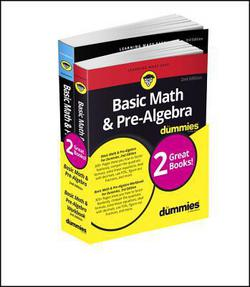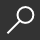Basic Math & Pre-Algebra for Dummies Book + Workbook Bundle
local_shippingFor DeliveryOn Order. Usually ships in 2-4 weeks
This item is Non-Returnable.
FREE Shipping for Club Members help### Overview

A 2-book bundle that adds up to success in basic math and pre-algebra

People of all ages -- from students in a math class to adults using math in the real world -- often dislike ever having to use arithmetic, fractions, decimals, percentages, geometry, and so on. Math is all around us, but it doesn't have to be hard. Basic Math & Pre-Algebra For Dummies and Basic Math & Pre-Algebra Workbook For Dummieswith Online Practice is a two-book bundle designed to help you improve your math skills whether you're a student tackling math homework or preparing for a test or an adult who wants to improve their own skills or help someone else learn to love numbers.

Basic Math & Pre-Algebra For Dummies provides mathematical and algebraic concepts into easy-to-understand sections. You'll learn to:

Master addition, subtraction, multiplication, and division

Wrangle the three E's of math: equations, expressions, and evaluation

Never fear a word problem again (even ones with fractions, decimals, and percents)

Know your prime numbers from composite numbers

Get your mind around factors and multiples

Learn the parts of a fraction and how to add, subtract, multiply, and divide fractions

Understand scientific notation and how to use it

Discover the X in algebra

And so much more

Basic Math & Pre-Algebra Workbook For Dummies covers negative numbers, fractions, decimals, geometry, graphing, basic algebra (and more), with plenty of problems so you can practice until your math is perfect. The workbook is divided into four sections, and you can work through each one or skip to any area you like:

Part 1 covers basic math and pre-algebra, including addition, subtraction, multiplication, and division; operations; negative numbers; and expressions.

Part 2 keeps things interesting with fractions, decimals, and percents.

Part 3 dives into headier topics, like scientific notation; weights and measures; geometry; and Xy-graphs.

Part 4 is all about algebraic expressions.

Each topic is briefly introduced, and you're shown how to solve the problems in that section. You can review sample questions with answers (showing how to solve the problem) and then complete practice problems on your own. Don't worry -- the answers are provided (with step-by-step details), so you can grade your work. Additionally, online practice comes free with this workbook, with extra practice questions corresponding with each chapter. The workbook itself has instruction on how to register to access and use the online practice.

Together these two books pack a powerful one-two punch. Grab this bundle to help you wrangle any math problem you encounter.

This item is Non-Returnable

• ISBN-13: 9781119387107
• ISBN-10: 1119387108
• Publisher: For Dummies
• Publish Date: June 2017
• Dimensions: 10 x 7.9 x 1.4 inches
• Shipping Weight: 2.15 pounds
• Page Count: 696